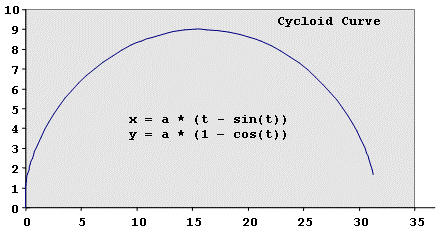# Reference: Parametric Curve

Model ElementReference_ParamCurve defines a parametric curve element. A parametric curve is defined in terms of one free parameter, u. Referring to the image below, assume a curve C is defined with respect to a coordinate system OXYZ.

## Description

The coordinates of any arbitrary P point on the curve, as measured in OXYZ, can be represented uniquely in terms of the free parameter u with three functions, f(u), g(u), and h(u), that define the x-, y- and z-coordinates of P. The extent of the curve is governed by the start and end values of u. This is a parametric representation for a curve.

Parametric curves may be open or closed. The curve is said to be closed when the closure boundary conditions shown below are satisfied:

f(u_start)       = f(u_end)
f'(u_start)      = f'(u_end)
f''(u_start)     = f''(u_end)
g(u_start)       = g(u_end)
g'(u_start)      = g'(u_end)
g''(u_start)     = g''(u_end)
h(u_start)       = h(u_end)
h'(u_start)      = h'(u_end)
h''(u_start)     = h''(u_end)

There are two types of parametric curves: analytical and spline. Spline representations require that (x,y,and z) data for the curve be provided in a matrix of data points or directly specified in the XML file. MotionSolve fits a spline through the data points and automatically parameterizes the curve in terms of a u variable.

Analytical representations require that the functions f(), g(), and h() are defined in a user-written subroutine CURSUB. The range of u needs to be explicitly specified for analytically defined curves.

When the curves are closed, MotionSolve requires that the first and last points of the spline data are the same. Similarly, you are required to satisfy the closure boundary conditions mentioned above.

Parametric curves are used to model higher pair constraints in MotionSolve. Reference_ParamCurve is only allowed to be defined on rigid bodies, not on flex bodies.

## Format

<Reference_ParamCurve
id       = "integer"
label               = "Name of the Curve"
is_u_closed         = { "TRUE" | "FALSE" }
is_curve_points     = { "TRUE" | "FALSE" }
[    quintic             = { "TRUE" | "FALSE" }       ]
{

matrix_id           = "integer" >
| u_start             = "real"
u_end               = "real"
usrsub_dll_name     = "valid_path_name"
usrsub_param_string  = "USER([[par_1[,...][,par_n]])"
usrsub_fnc_name     = "custom_fnc_name" >
| u_start             = "real"
u_end               = "real"
script_name         = valid_path_name
interpreter         = "PYTHON" | "MATLAB"
usrsub_param_string  = "USER([[par_1[,...][,par_n]])"
usrsub_fnc_name     = "custom_fnc_name" >
| num_xyz_triple      = "integer" >
x1    y1     z1
x2    y2     z2
...  ...    ...
xn    yn     zn
}
/>

## Attributes

id
Element identification number (integer>0). This number is unique to all Reference_ParamCurves.
label
The name of the Reference_ParamCurve elements.
is_u_closed
Select from TRUE or FALSE. If the curve is closed in the U parametric space, select TRUE. If the curve is open in the U parametric space, select FALSE.
is_curve_points

Select from TRUE or FALSE.

TRUE means that the curve passes through the points.

FALSE means that the curve (B-spline) stays close to the points, but does not pass through them in general.

quintic

Select from TRUE or FALSE.

TRUE indicates that the order of curve interpolation is 5

FALSE indicates that the order of curve interpolation is 4

Default value is FALSE.

matrix_id
Specifies the Reference_Matrix element that contains the curve data. The matrix should contain only the x-, y-, and z- data for the individual curve points. For a close curve, the first and last data points must be the same.
u_start
The minimum value of U. Note that u_start < u_end. Set u_start only when is_curve_points = FALSE. In other words, if a CURSUB is being used to define the curve.
u_end
The maximum value of U. Note that u_start < u_end. Set u_end only when is_curve_points = FALSE. In other words, if a CURSUB is being used to define the curve.
usrsub_param_string
The list of parameters that are passed from the data file to the user defined CURSUB. Use this keyword only when is_curve_points = FALSE. In other words, a CURSUB is being used to define the curve.
usrsub_dll_name
Specifies the path and name of the DLL or shared library containing user subroutines. MotionSolve uses this information to load the user subroutine CURSUB in the DLL at run time. Use this keyword only if is_curve_points = FALSE. In other words, if a CURSUB is being used to define the curve.
usrsub_fnc_name
Specifies an alternative name for the user subroutine CURSUB.
script_name
Specifies the path and name of the user written script that contains the routine specified by usrsub_fnc_name.
interpreter
Specifies the interpreted language that the user script is written in. Valid choices are MATLAB or PYTHON.
num_xyz_triple

Specifies the number of x-y-z points in the curve. Use only when the curve points are embedded in the Reference_ParamCurve element itself. This option cannot be used with MATRIX or usrsub_param_string parameters.

## Example

The following example defines how to implement a cycloid that is defined in parametric space in MotionSolve. The independent parameter for this example is the variable t. The cycloid is defined as:

x = a * (t - sin(t))
y = a * (1-cos(t))
The cycloid is shown in the image below. The statement defining the cycloid described above has the following curve attributes:
• The ID of the Reference_Curve is 569.
• It defines an open curve.
• The independent parameter t starts at zero.
• The independent parameter t ends at 5.1.
• The curve points are specified in matrix_id/23.The corresponding MotionSolve XML statement is:

<Reference_ParamCurve
id                  = "569"
is_u_closed         = "FALSE"
is_curve_points     = "TRUE"
matrix_id           = "23"
/>

The second example shows how to specify exactly the same for implementation in a user defined subroutine CURSUB.

<Reference_ParamCurve
id                  = "569"
is_u_closed         = "FALSE"
is_curve_points     = "FALSE"
u_start             = "0"
u_end               = "5.1"
usrsub_param_string = "USER(22,11)"
usrsub_dll_name     = "C:\gates\curve.dll"
/>